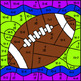# Super Bowl Mystery Picture ~ Addition & Subtraction Within 20 ~ FootballSubject
Resource Type
File Type

PDF

(1 MB|4 pages)
Standards
• Product Description
• StandardsNEW

First grade students are expected to add and subtract within 20, demonstrating fluency for addition and subtraction within 10. This activity allows the student practice in becoming fluent with these math facts.

Included is a student sheet and key.

Click here for more Mystery Picture ~ Color by Code activities. You'll find activities for both math and phonics. Check them out!

If you like this activity, I encourage you to follow my store so you'll be notified of new or free products as they are added to my store.

As always, I appreciate your feedback and you'll receive TPT points to use on future purchases.

Thank you!

Fluently add and subtract within 20 using mental strategies. By end of Grade 2, know from memory all sums of two one-digit numbers.
Add and subtract within 20, demonstrating fluency for addition and subtraction within 10. Use strategies such as counting on; making ten (e.g., 8 + 6 = 8 + 2 + 4 = 10 + 4 = 14); decomposing a number leading to a ten (e.g., 13 - 4 = 13 - 3 - 1 = 10 - 1 = 9); using the relationship between addition and subtraction (e.g., knowing that 8 + 4 = 12, one knows 12 - 8 = 4); and creating equivalent but easier or known sums (e.g., adding 6 + 7 by creating the known equivalent 6 + 6 + 1 = 12 + 1 = 13).
Total Pages
4 pages
Included
Teaching Duration
N/A
Report this Resource to TpT
Reported resources will be reviewed by our team. Report this resource to let us know if this resource violates TpT’s content guidelines.